### 1 引言

$\text{lr_rate}={d}_{model}^{-0.5}\cdot \text{min}\left({\text{step_num}}^{-0.5},\text{step_num}\cdot {\text{warmup_steps}}^{-1.5}\right)\phantom{\rule{0.278em}{0ex}}\phantom{\rule{0.278em}{0ex}}\phantom{\rule{0.278em}{0ex}}\phantom{\rule{0.278em}{0ex}}\phantom{\rule{0.278em}{0ex}}\phantom{\rule{0.278em}{0ex}}\phantom{\rule{0.278em}{0ex}}\left(1\right)$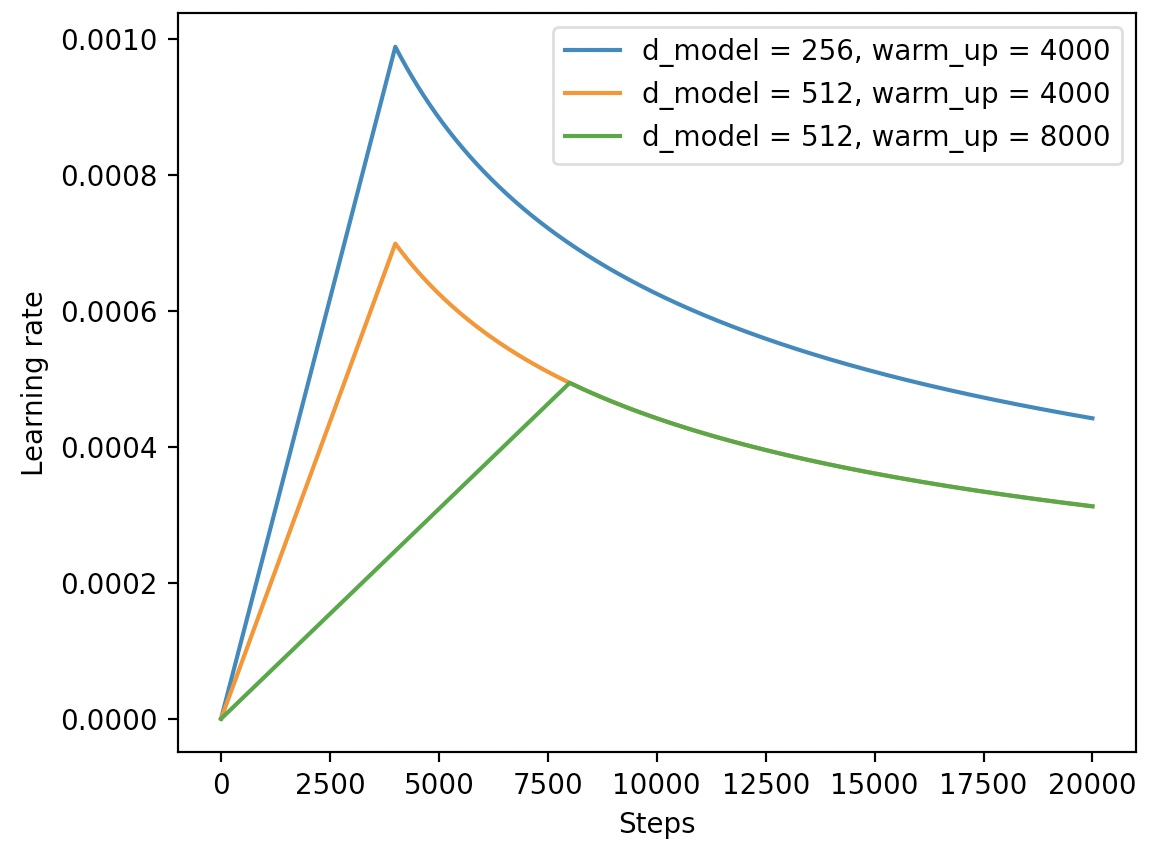xxxxxxxxxx271class CustomSchedule(object):2    def __init__(self, d_model, warmup_steps=4000, optimizer=None):3        super(CustomSchedule, self).__init__()4        self.d_model = torch.tensor(d_model, dtype=torch.float32)5        self.warmup_steps = warmup_steps6        self.steps = 1.7        self.optimizer = optimizer8
9    def step(self):10        arg1 = self.steps ** -0.511        arg2 = self.steps * (self.warmup_steps ** -1.5)12        self.steps += 1.13        lr = (self.d_model ** -0.5) * min(arg1, arg2)14        for p in self.optimizer.param_groups:15            p['lr'] = lr16        return lr17
18def train(config):19    optimizer = torch.optim.Adam(translation_model.parameters(),20                                 lr=0.,21                                 betas=(config.beta1, config.beta2), eps=config.epsilon)22    lr_scheduler = CustomSchedule(config.d_model, optimizer=optimizer)23    ...24    loss.backward()25    lr_scheduler.step()26    optimizer.step()27    ...

### 2 学习率动态调整

xxxxxxxxxx11from transformers import optimization

optimization模块中，一共包含了6种常见的学习率动态调整方式，包括constant、constant_with_warmup、linear、polynomial、cosine 和cosine_with_restarts，其分别通过一个函数来返回对应的实例化对象。

#### 2.1 constant

optimization模块中可以通过get_constant_schedule函数来返回对应的常数动态学习率调整方法。顾名思义，常数学习率动态调整就是学习率是一个恒定不变的常数，也就是说相当于没用。为了方便后续对学习率的变化进行可视化，这里我们先随便定义一个网络模型，代码如下：

xxxxxxxxxx111import torch2import torch.nn as nn3
4class Model(nn.Module):5    def __init__(self):6        super(Model, self).__init__()7        self.fc = nn.Linear(5, 10)8
9    def forward(self, x):10        out = self.fc(x).sum()11        return out

xxxxxxxxxx171from transformers import optimization2
3if __name__ == '__main__':4    x = torch.rand([8, 5])5    model = Model()6    model.train()7    steps = 10008    optimizer = torch.optim.Adam(model.parameters(), lr=1.0)9    scheduler = optimization.get_constant_schedule(optimizer, last_epoch=-1)10    lrs = []11    for _ in range(steps):12        loss = model(x)13        optimizer.zero_grad()14        loss.backward()15        optimizer.step()16        scheduler.step()17        lrs.append(scheduler.get_last_lr()) 

xxxxxxxxxx41    plt.figure(figsize=(7, 4))2    plt.plot(range(steps), lrs, label=name)3    plt.legend(fontsize=13)4    plt.show()#### 2.2 constant_with_warmup

optimization模块中可以通过get_constant_schedule_with_warmup函数来返回对应的动态学习率调整的实例化方法。从名字可以看出，该方法最终得到的是一个带warmup的常数学习率变化。在模型训练的过程中，我们可以通过以下方式来进行使用：

xxxxxxxxxx11scheduler = optimization.get_constant_schedule_with_warmup(optimizer, num_warmup_steps=300)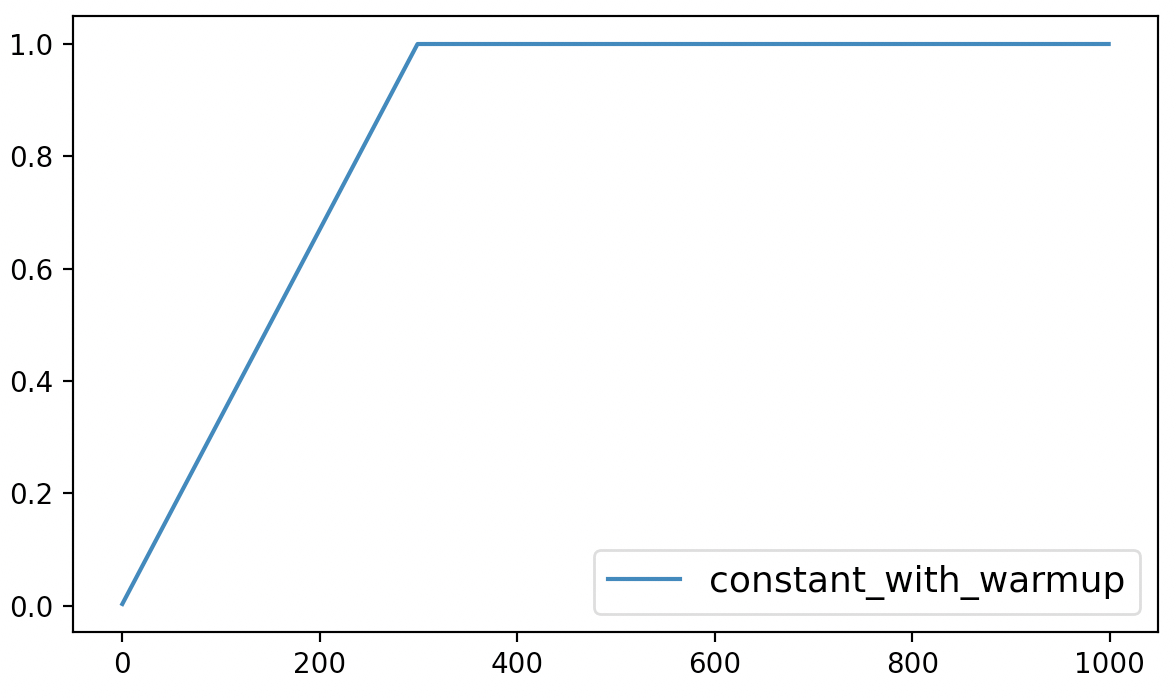#### 2.3 linear

optimization模块中可以通过get_constant_schedule_with_warmup函数来返回对应的动态学习率调整的实例化方法。从名字可以看出，该方法最终得到的是一个带warmup的常数学习率变化。在模型训练的过程中，我们可以通过以下方式来进行使用：

xxxxxxxxxx31scheduler = optimization.get_linear_schedule_with_warmup(optimizer,2                                                         num_warmup_steps=300,3                                                         num_training_steps=steps)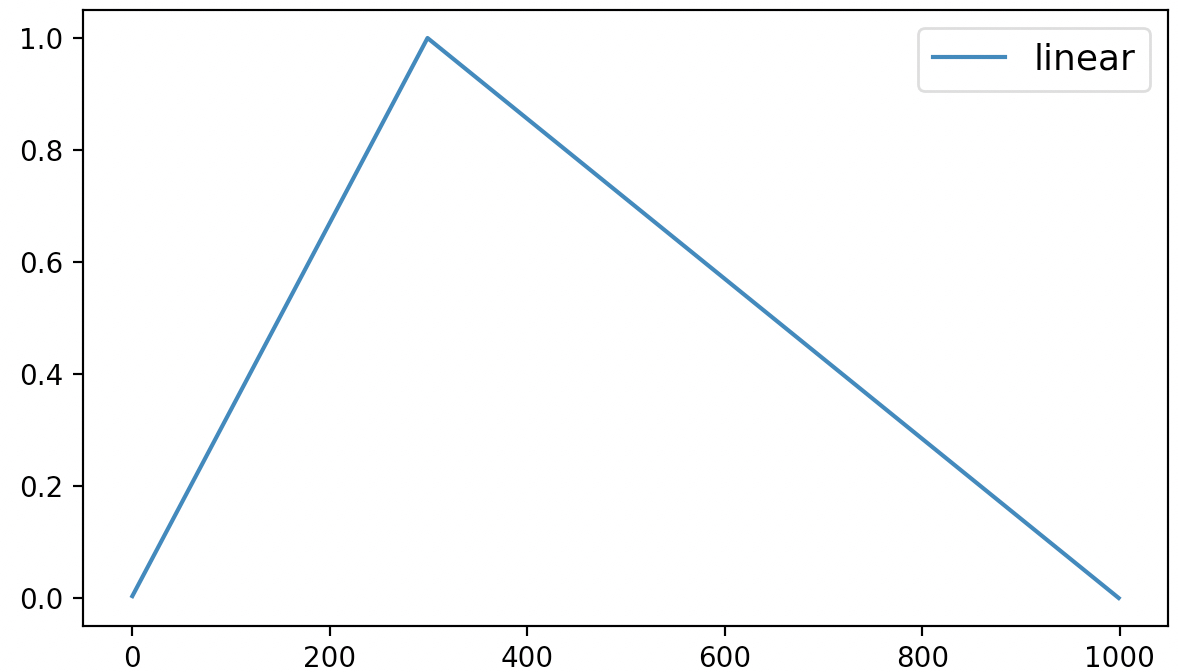#### 2.4 polynomial

optimization模块中可以通过get_constant_schedule_with_warmup函数来返回对应的动态学习率调整的实例化方法。从名字可以看出，该方法最终得到的是一个基于多项式的学习率动态调整策略。在模型训练的过程中，我们可以通过以下方式来进行使用：

xxxxxxxxxx51scheduler = optimization.get_polynomial_decay_schedule_with_warmup(optimizer,2                                                                   num_warmup_steps=300,3                                                                   num_training_steps=steps,4                                                                   lr_end = 1e-7,5                                                                   power=3)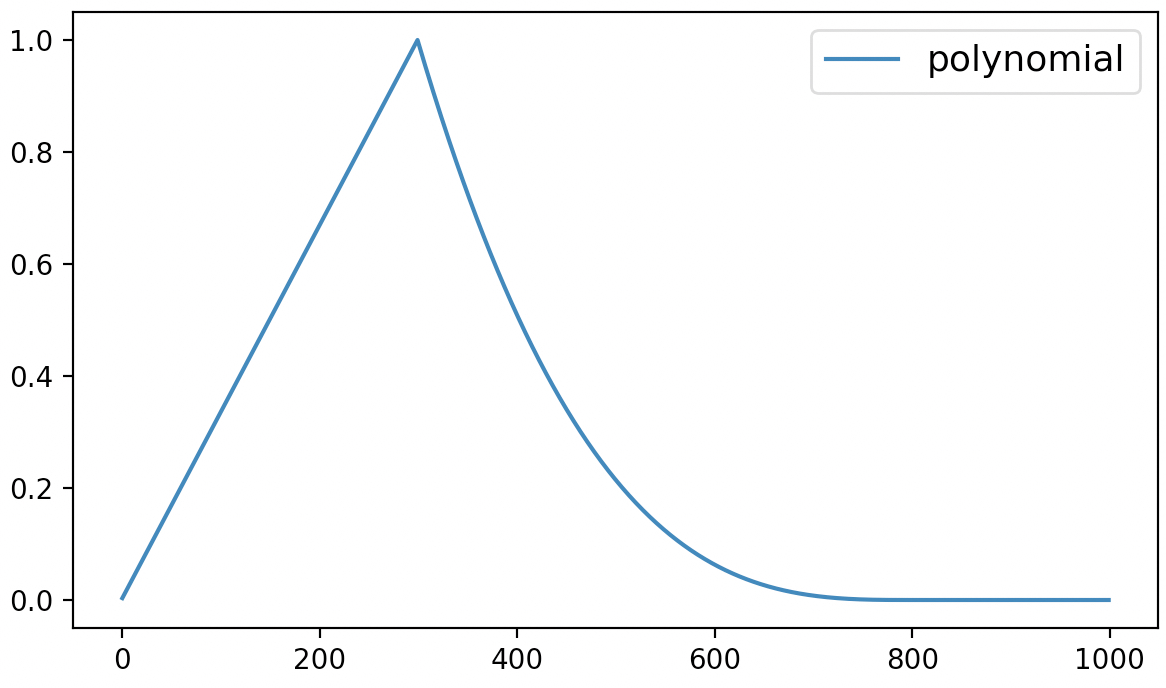#### 2.5 cosine

optimization模块中可以通过get_cosine_schedule_with_warmup来返回基于cosine函数的动态学习率调整方法。在模型训练过程中我们可以通过如下方式来进行调用：

xxxxxxxxxx41scheduler = optimization.get_cosine_schedule_with_warmup(optimizer,2                                                         num_warmup_steps=300,3                                                         num_training_steps=steps,4                                                         num_cycles=2)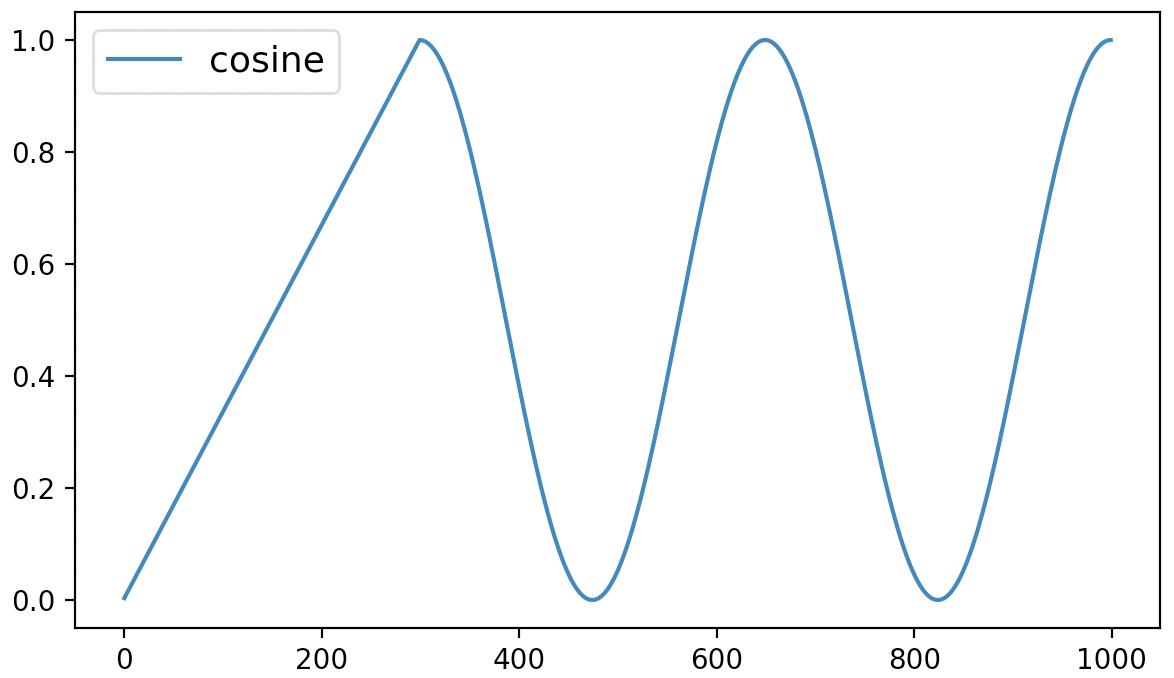#### 2.6 cosine_with_restarts

optimization模块中可以通过get_cosine_with_hard_restarts_schedule_with_warmup来返回基于cosine函数的硬重启动态学习率调整方法。所谓硬重启就是学习率衰减到0之后直接变回到最大值的方式。在模型训练过程中我们可以通过如下方式来进行调用：

xxxxxxxxxx41scheduler = optimization.get_cosine_with_hard_restarts_schedule_with_warmup(optimizer,2                                                                            num_warmup_steps=300,3                                                                            num_training_steps=steps,4                                                                            num_cycles=2)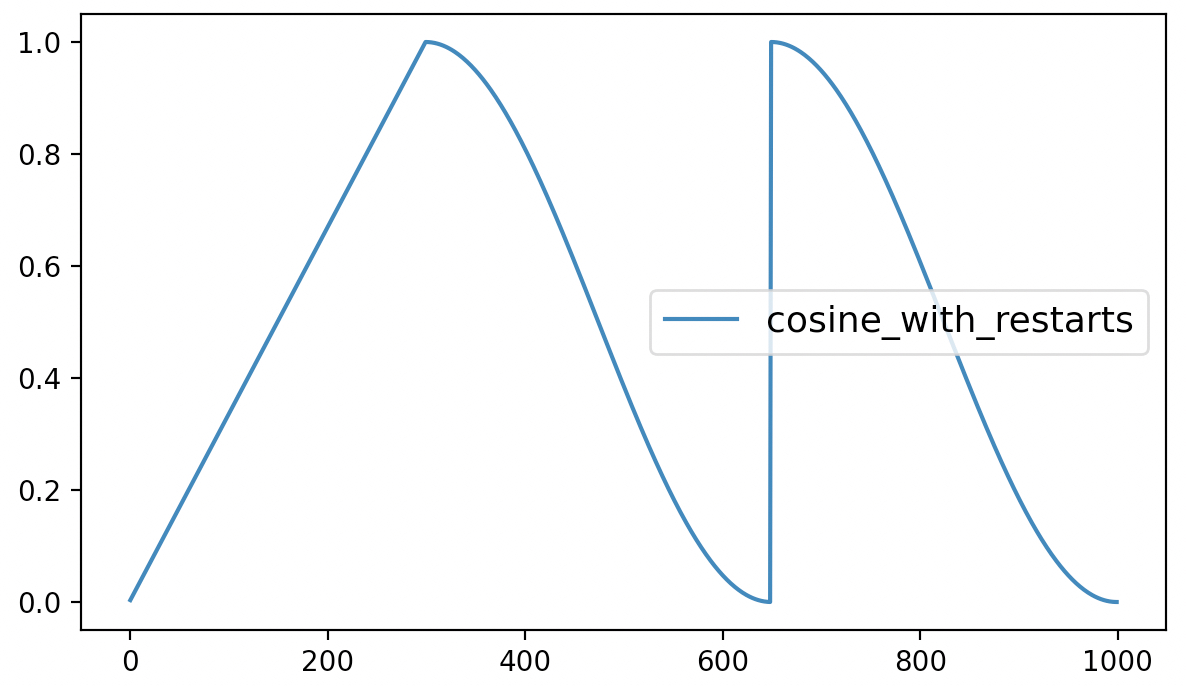#### 2.7 get_scheduler

xxxxxxxxxx61from transformers import get_scheduler2def get_scheduler(3    name: Union[str, SchedulerType],4    optimizer: Optimizer,5    num_warmup_steps: Optional[int] = None,6    num_training_steps: Optional[int] = None):

xxxxxxxxxx61scheduler = optimization.get_cosine_with_hard_restarts_schedule_with_warmup(optimizer,2                                                                            num_warmup_steps=300,3                                                                            num_training_steps=steps,4                                                                            num_cycles=1)5scheduler = get_scheduler(name="cosine_with_restarts", optimizer=optimizer,6                          num_warmup_steps=300, num_training_steps=steps)

### 3 学习率调整实现

xxxxxxxxxx41from torch.optim.lr_scheduler import LambdaLR2class LambdaLR(_LRScheduler):3    def __init__(self, optimizer, lr_lambda, last_epoch=-1):4        pass

#### 3.1 constant实现

xxxxxxxxxx21def get_constant_schedule(Optimizer, last_epoch = -1):2    return LambdaLR(optimizer, lambda _: 1, last_epoch=last_epoch)

#### 3.2 constant_with_warmup实现

$\begin{array}{c}\text{lr_coef}=\left\{\begin{array}{ll}\frac{\text{current_step}}{\text{num_warmup_steps}},& \text{current_step}\phantom{\rule{0.278em}{0ex}}<\phantom{\rule{0.278em}{0ex}}\text{num_warmup_steps}\\ 1.0,& \text{current_step}\phantom{\rule{0.278em}{0ex}}\ge \phantom{\rule{0.278em}{0ex}}\text{num_warmup_steps}\end{array}\phantom{\rule{0.278em}{0ex}}\phantom{\rule{0.278em}{0ex}}\phantom{\rule{0.278em}{0ex}}\phantom{\rule{0.278em}{0ex}}\phantom{\rule{0.278em}{0ex}}\phantom{\rule{0.278em}{0ex}}\phantom{\rule{0.278em}{0ex}}\left(2\right)\end{array}$

xxxxxxxxxx61def get_constant_schedule_with_warmup(Optimizer, num_warmup_steps,last_epoch = -1):2    def lr_lambda(current_step):3        if current_step < num_warmup_steps:4            return float(current_step) / float(max(1.0, num_warmup_steps))5        return 1.06    return LambdaLR(optimizer, lr_lambda, last_epoch=last_epoch)

#### 3.3 linear实现

$\begin{array}{c}\text{lr_coef}=\left\{\begin{array}{ll}\frac{\text{current_step}}{\text{num_warmup_steps}},& \text{current_step}\phantom{\rule{0.278em}{0ex}}<\phantom{\rule{0.278em}{0ex}}\text{num_warmup_steps}\\ \frac{\text{num_training_steps}-\text{current_step}}{\text{num_training_steps}-\text{num_warmup_steps}},& \text{current_step}\phantom{\rule{0.278em}{0ex}}\ge \phantom{\rule{0.278em}{0ex}}\text{num_warmup_steps}\end{array}\phantom{\rule{0.278em}{0ex}}\phantom{\rule{0.278em}{0ex}}\phantom{\rule{0.278em}{0ex}}\phantom{\rule{0.278em}{0ex}}\phantom{\rule{0.278em}{0ex}}\phantom{\rule{0.278em}{0ex}}\phantom{\rule{0.278em}{0ex}}\left(3\right)\end{array}$

xxxxxxxxxx71def get_linear_schedule_with_warmup(optimizer, num_warmup_steps, num_training_steps, last_epoch=-1):2    def lr_lambda(current_step: int):3        if current_step < num_warmup_steps:4            return float(current_step) / float(max(1, num_warmup_steps))5        return max( 0.0, float(num_training_steps - current_step) / 6          float(max(1, num_training_steps - num_warmup_steps)))7    return LambdaLR(optimizer, lr_lambda, last_epoch)

#### 3.4 polynomial实现

$\begin{array}{c}\text{lr_coef}=\left\{\begin{array}{ll}\frac{\text{current_step}}{\text{num_warmup_steps}},& \text{current_step}\phantom{\rule{0.278em}{0ex}}<\phantom{\rule{0.278em}{0ex}}\text{num_warmup_steps}\\ \frac{\text{lr_end}}{\text{lr_init}},& \text{current_step}\phantom{\rule{0.278em}{0ex}}>\phantom{\rule{0.278em}{0ex}}\text{num_training_steps}\\ \frac{\left(\text{lr_init}-\text{lr_end}\right)\cdot {\left[1-\frac{\text{current_step}-\text{num_warmup_steps}}{\text{num_training_steps}-\text{num_warmup_steps}}\right]}^{\text{power}}+\text{lr_end}}{\text{lr_init}},& \text{num_warmup_steps}\phantom{\rule{0.278em}{0ex}}\le \phantom{\rule{0.278em}{0ex}}\text{current_step}\phantom{\rule{0.278em}{0ex}}\le \phantom{\rule{0.278em}{0ex}}\text{num_training_steps}\end{array}\phantom{\rule{0.278em}{0ex}}\phantom{\rule{0.278em}{0ex}}\phantom{\rule{0.278em}{0ex}}\phantom{\rule{0.278em}{0ex}}\phantom{\rule{0.278em}{0ex}}\phantom{\rule{0.278em}{0ex}}\phantom{\rule{0.278em}{0ex}}\left(4\right)\end{array}$

xxxxxxxxxx161def get_polynomial_decay_schedule_with_warmup(optimizer, 2            num_warmup_steps, num_training_steps, lr_end=1e-7, power=1.0, last_epoch=-1):3    lr_init = optimizer.defaults["lr"]4    assert lr_init > lr_end, f"lr_end ({lr_end}) must be be smaller than initial lr ({lr_init})"5    def lr_lambda(current_step):6        if current_step < num_warmup_steps:7            return float(current_step) / float(max(1, num_warmup_steps))8        elif current_step > num_training_steps:9            return lr_end / lr_init  # as LambdaLR multiplies by lr_init10        else:11            lr_range = lr_init - lr_end12            decay_steps = num_training_steps - num_warmup_steps13            pct_remaining = 1 - (current_step - num_warmup_steps) / decay_steps14            decay = lr_range * pct_remaining ** power + lr_end15            return decay / lr_init  # as LambdaLR multiplies by lr_init16    return LambdaLR(optimizer, lr_lambda, last_epoch)

#### 3.5 cosine实现

$\begin{array}{c}\text{lr_coef}=\left\{\begin{array}{ll}\frac{\text{current_step}}{\text{num_warmup_steps}},& \text{current_step}\phantom{\rule{0.278em}{0ex}}<\phantom{\rule{0.278em}{0ex}}\text{num_warmup_steps}\\ \frac{1}{2}\cdot \left(1+\mathrm{cos}\left(2\cdot \pi \cdot \text{num_cycles}\cdot \frac{\text{current_step}-\text{num_warmup_steps}}{\text{num_training_steps}-\text{num_warmup_steps}}\right)\right),& \text{current_step}\phantom{\rule{0.278em}{0ex}}\ge \phantom{\rule{0.278em}{0ex}}\text{num_warmup_steps}\end{array}\phantom{\rule{0.278em}{0ex}}\phantom{\rule{0.278em}{0ex}}\phantom{\rule{0.278em}{0ex}}\phantom{\rule{0.278em}{0ex}}\phantom{\rule{0.278em}{0ex}}\phantom{\rule{0.278em}{0ex}}\phantom{\rule{0.278em}{0ex}}\left(5\right)\end{array}$

xxxxxxxxxx91def get_cosine_schedule_with_warmup(optimizer, num_warmup_steps,2                            num_training_steps, num_cycles = 0.5, last_epoch = -1):3    def lr_lambda(current_step):4        if current_step < num_warmup_steps:5            return float(current_step) / float(max(1, num_warmup_steps))6        progress = float(current_step - num_warmup_steps) / 7                   float(max(1, num_training_steps - num_warmup_steps))8        return max(0.0, 0.5 * (1.0 + math.cos(math.pi * float(num_cycles) * 2.0 * progress)))9    return LambdaLR(optimizer, lr_lambda, last_epoch)

#### 3.6 cosine_with_restarts实现

$\begin{array}{c}\text{lr_coef}=\left\{\begin{array}{ll}\frac{\text{current_step}}{\text{num_warmup_steps}},& \text{current_step}\phantom{\rule{0.278em}{0ex}}<\phantom{\rule{0.278em}{0ex}}\text{num_warmup_steps}\\ 0.0,& \text{current_step}\phantom{\rule{0.278em}{0ex}}>\phantom{\rule{0.278em}{0ex}}\text{num_training_steps}\\ \frac{1}{2}\cdot \left(1+\mathrm{cos}\left(\pi \cdot \left(\text{num_cycles}\cdot \frac{\text{current_step}-\text{num_warmup_steps}}{\text{num_training_steps}-\text{num_warmup_steps}}\right)\mathrm{%}1.0\right)\right),& \text{num_warmup_steps}\phantom{\rule{0.278em}{0ex}}\le \phantom{\rule{0.278em}{0ex}}\text{current_step}\phantom{\rule{0.278em}{0ex}}\le \phantom{\rule{0.278em}{0ex}}\text{num_training_steps}\end{array}\phantom{\rule{0.278em}{0ex}}\phantom{\rule{0.278em}{0ex}}\phantom{\rule{0.278em}{0ex}}\phantom{\rule{0.278em}{0ex}}\phantom{\rule{0.278em}{0ex}}\phantom{\rule{0.278em}{0ex}}\phantom{\rule{0.278em}{0ex}}\left(6\right)\end{array}$

xxxxxxxxxx111def get_cosine_with_hard_restarts_schedule_with_warmup(optimizer, num_warmup_steps, 2                  num_training_steps, num_cycles = 1, last_epoch  = -1):3    def lr_lambda(current_step):4        if current_step < num_warmup_steps:5            return float(current_step) / float(max(1, num_warmup_steps))6        progress = float(current_step - num_warmup_steps) / 7                   float(max(1, num_training_steps - num_warmup_steps))8        if progress >= 1.0:9            return 0.010        return max(0.0, 0.5 * (1.0 + math.cos(math.pi * ((float(num_cycles) * progress) % 1.0))))11    return LambdaLR(optimizer, lr_lambda, last_epoch)

#### 3.7 transfromer实现

xxxxxxxxxx81def get_customized_schedule_with_warmup(optimizer, num_warmup_steps, d_model=1.0, last_epoch=-1):2    def lr_lambda(current_step):3        current_step += 14        arg1 = current_step ** -0.55        arg2 = current_step * (num_warmup_steps ** -1.5)6        return (d_model ** -0.5) * min(arg1, arg2)7
8    return LambdaLR(optimizer, lr_lambda, last_epoch)

xxxxxxxxxx41optimizer = torch.optim.Adam(model.parameters(), lr=1.0)2scheduler = get_customized_schedule_with_warmup(optimizer,3                                                num_warmup_steps=200,4                                                d_model=728)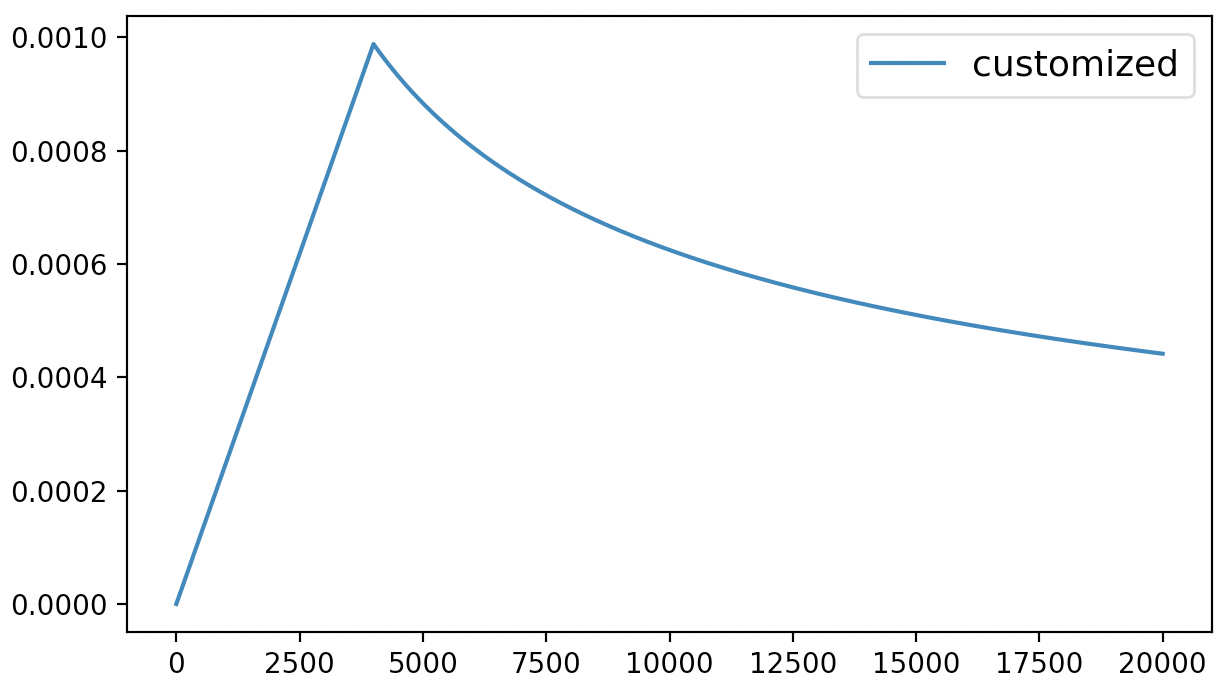### 4 LambdaLR原理

#### 4.1 实现逻辑

xxxxxxxxxx421class _LRScheduler(object):2
3    def __init__(self, optimizer, last_epoch=-1):4        if last_epoch == -1:5            for group in optimizer.param_groups:6                group.setdefault('initial_lr', group['lr'])7        ......8        self.base_lrs = list(map(lambda group: group['initial_lr'], optimizer.param_groups))9        self.last_epoch = last_epoch10        ......11        self.step()12
13    def get_lr(self):14        raise NotImplementedError15
16    def step(self, epoch=None):17        ......18        self._step_count += 119        with _enable_get_lr_call(self):20            if epoch is None:21                self.last_epoch += 122                values = self.get_lr()23            else:24                self.last_epoch = epoch25                if hasattr(self, "_get_closed_form_lr"):26                    values = self._get_closed_form_lr()27                else:28                    values = self.get_lr()29        for param_group, lr in zip(self.optimizer.param_groups, values):30            param_group['lr'] = lr31        ......32
33class LambdaLR(_LRScheduler):34  35    def __init__(self, optimizer, lr_lambda, last_epoch=-1):36        self.optimizer = optimizer37        self.last_epoch = last_epoch38        super(LambdaLR, self).__init__(optimizer, last_epoch)39
40    def get_lr(self):41        return [base_lr * lmbda(self.last_epoch)42                for lmbda, base_lr in zip(self.lr_lambdas, self.base_lrs)]

xxxxxxxxxx41for epoch in epoches:2    for data in data_iter:3        optimizer.step()4        scheduler.step(epoch=epoch)

#### 4.2 学习率恢复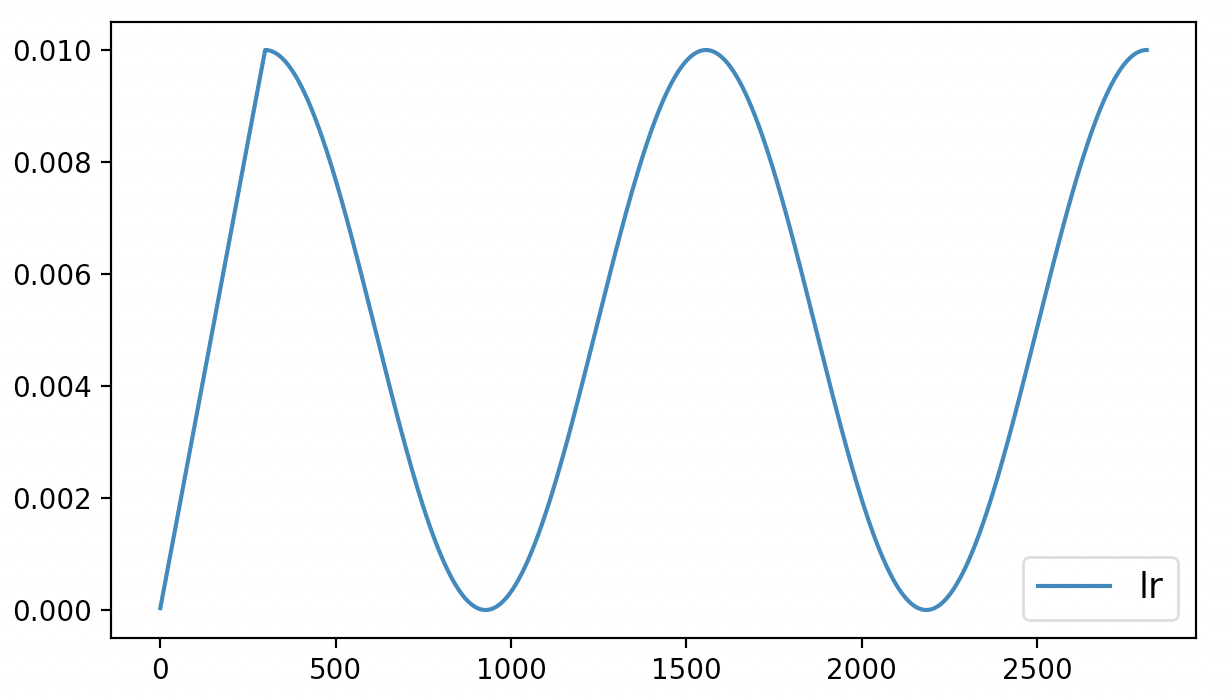xxxxxxxxxx241        last_epoch = -12        if os.path.exists('./model.pt'):3            checkpoint = torch.load('./model.pt')4            last_epoch = checkpoint['last_epoch']5            self.model.load_state_dict(checkpoint['model_state_dict'])6
7        num_training_steps = len(train_iter) * self.epochs8        optimizer = torch.optim.Adam([{"params": self.model.parameters(),9                                       "initial_lr": self.learning_rate}])10        scheduler = get_cosine_schedule_with_warmup(optimizer, num_warmup_steps=300,11                                                    num_training_steps=num_training_steps,12                                                    num_cycles=2, last_epoch=last_epoch)13        for epoch in range(self.epochs):14            for i, (x, y) in enumerate(train_iter):15                loss, logits = self.model(x, y)16                optimizer.zero_grad()17                loss.backward()18                optimizer.step()  # 执行梯度下降19                scheduler.step()20                lrs.append(scheduler.get_last_lr())21        ......22        torch.save({'last_epoch': scheduler.last_epoch,23                    'model_state_dict': self.model.state_dict()},24                   './model.pt')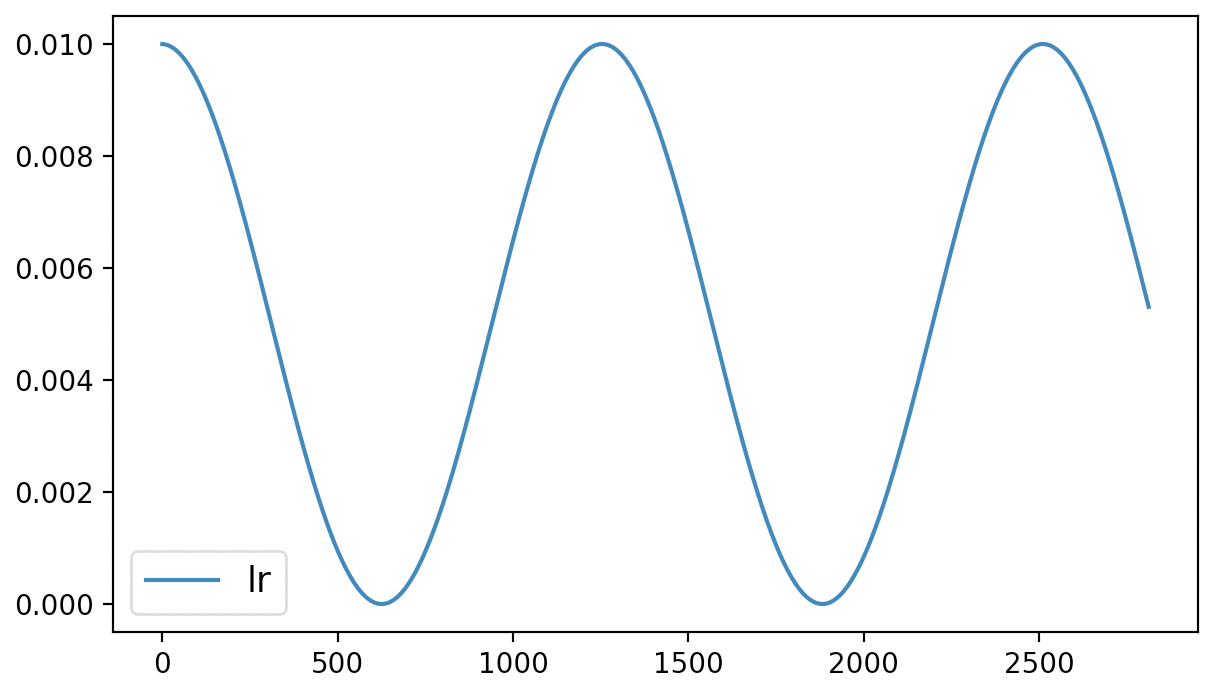### 推荐阅读

 如何用@修饰器来缓存数据预处理结果？

 Pytorch中模型的保存与迁移

 训练模型时如何便捷保存日志？